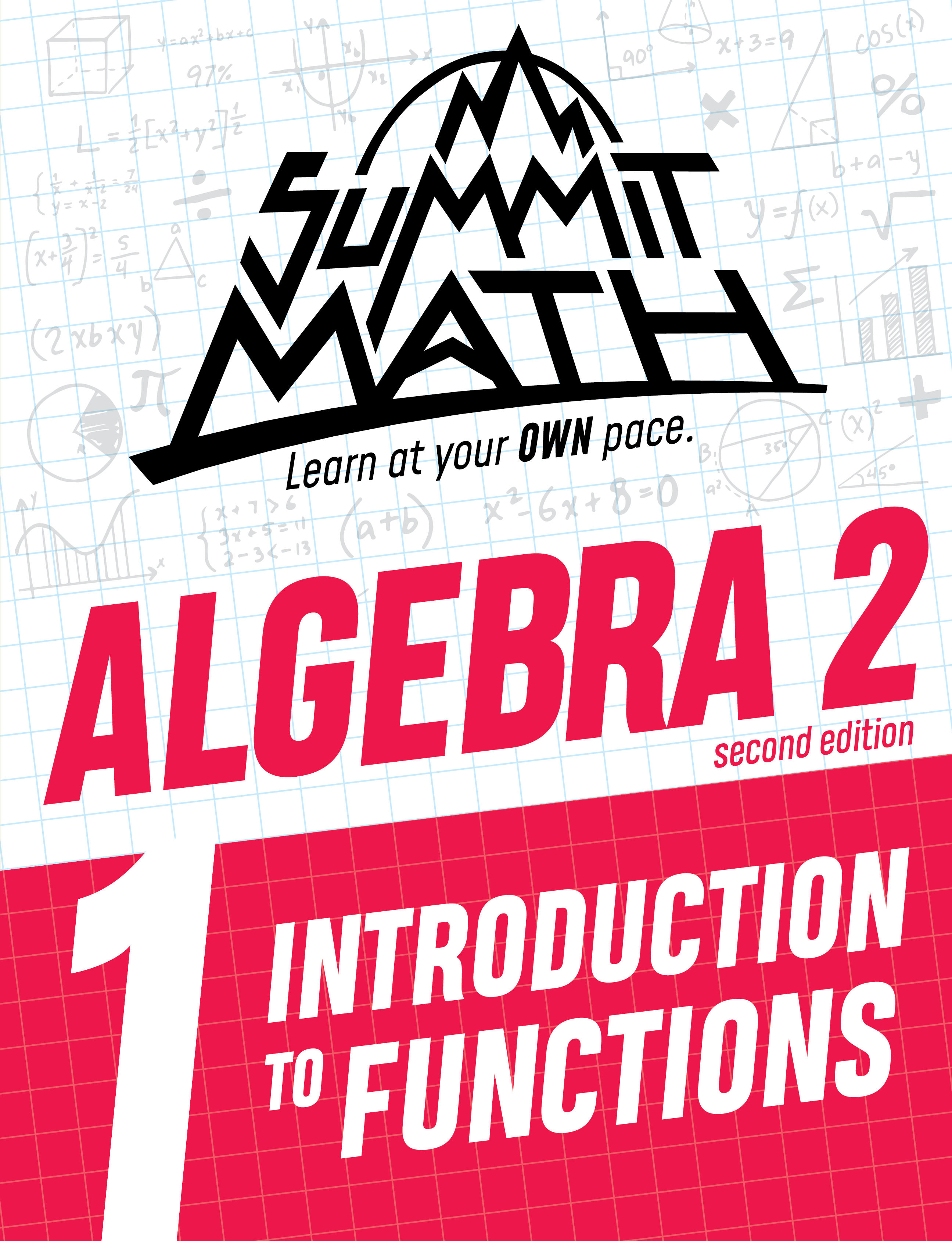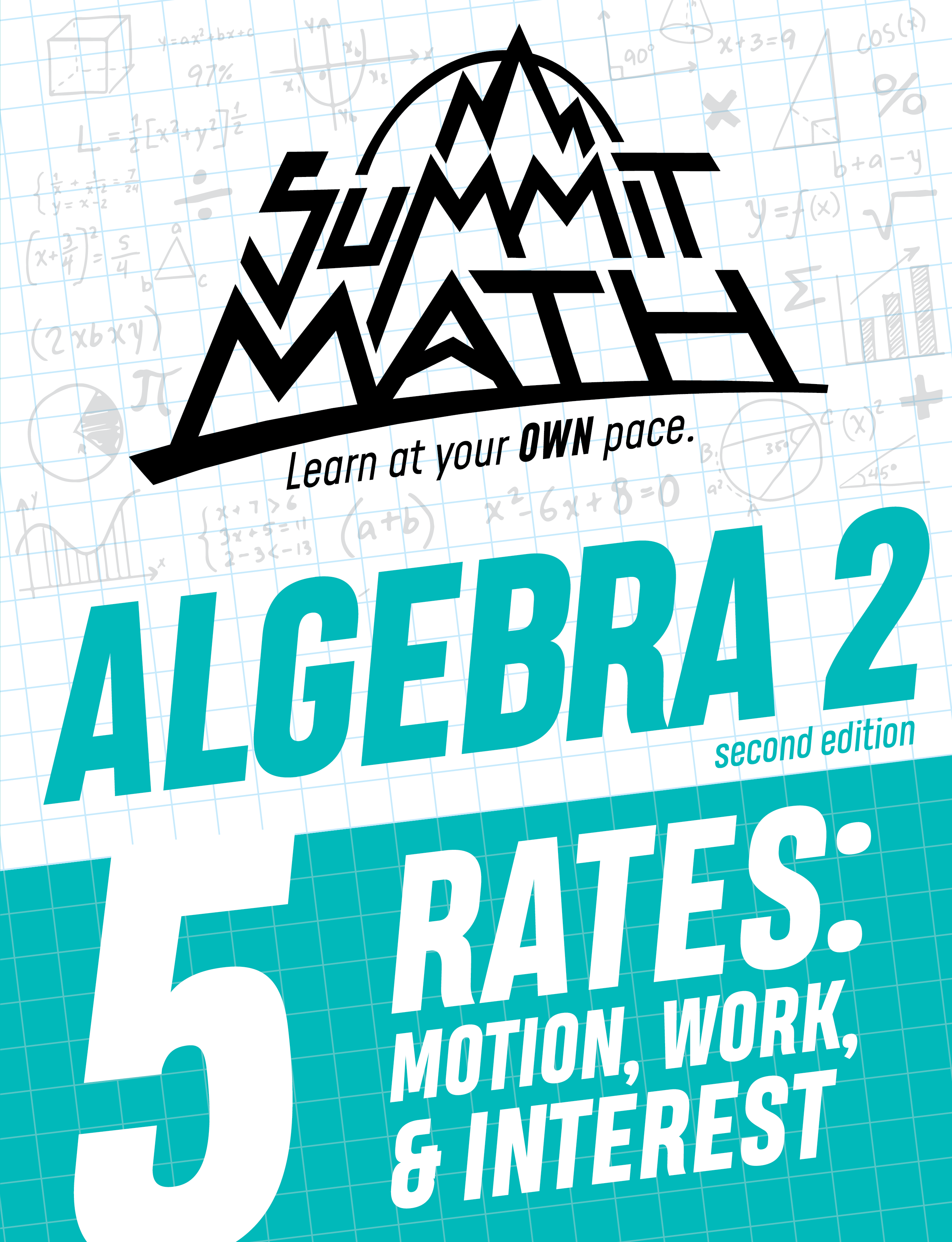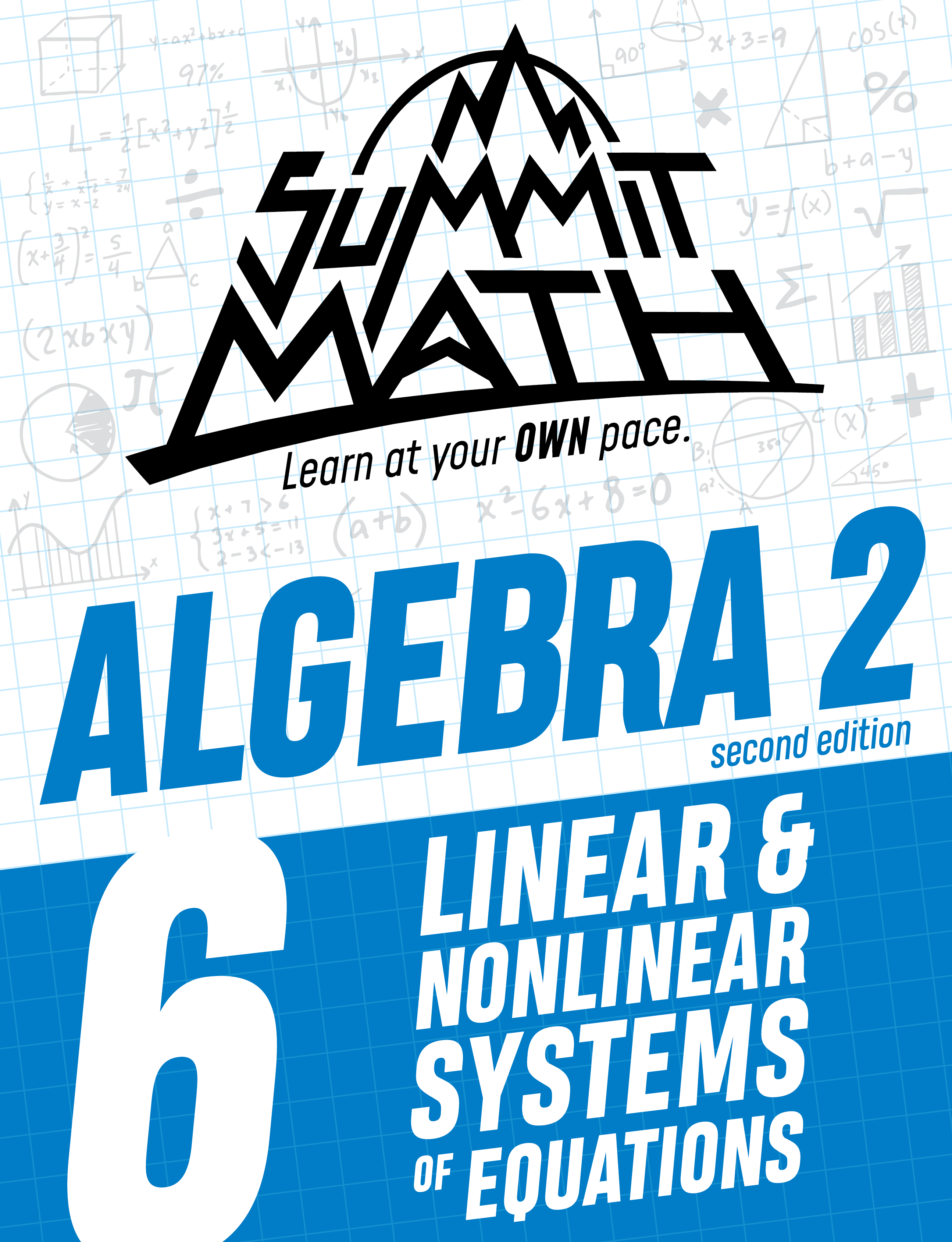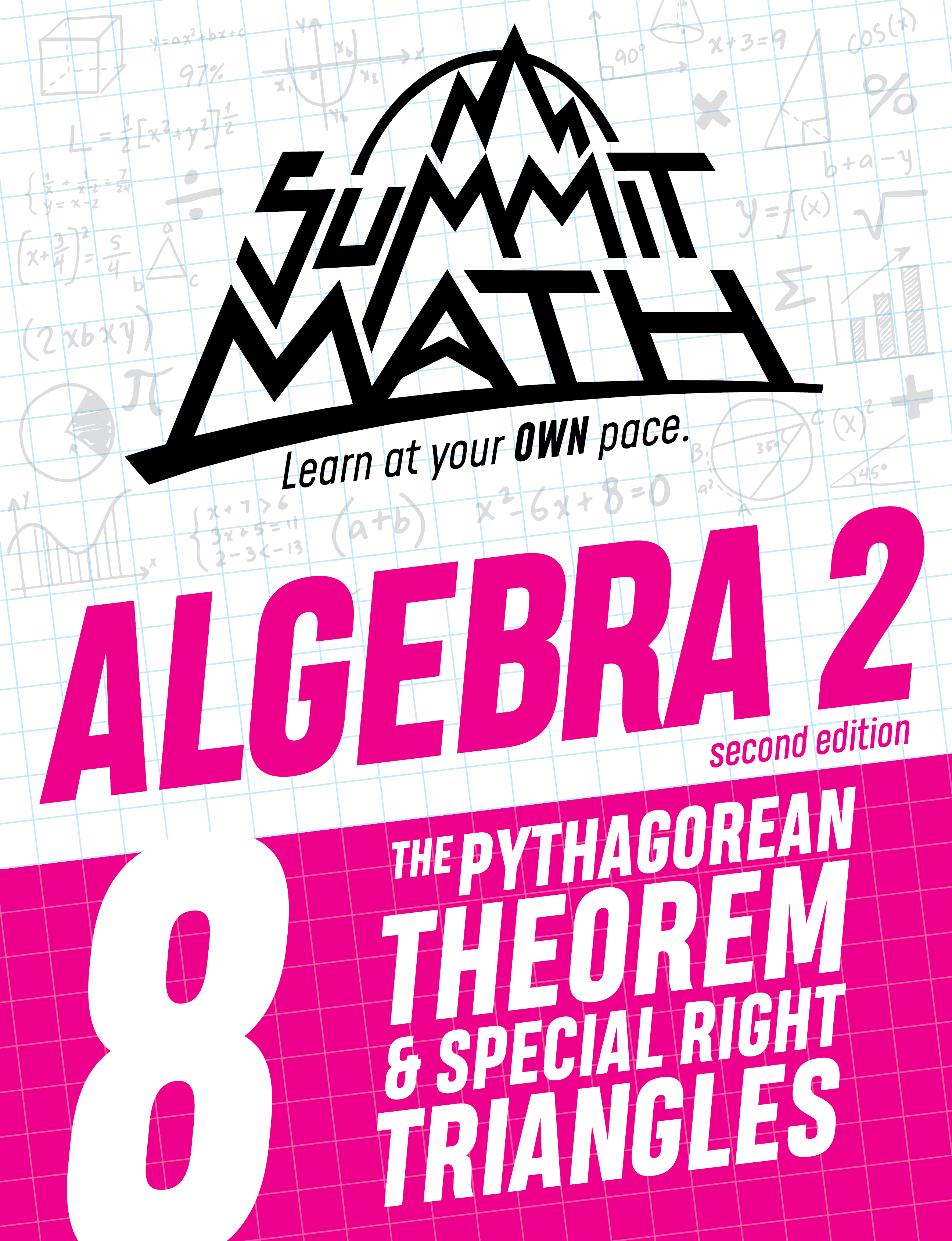## 1,900+ scenarios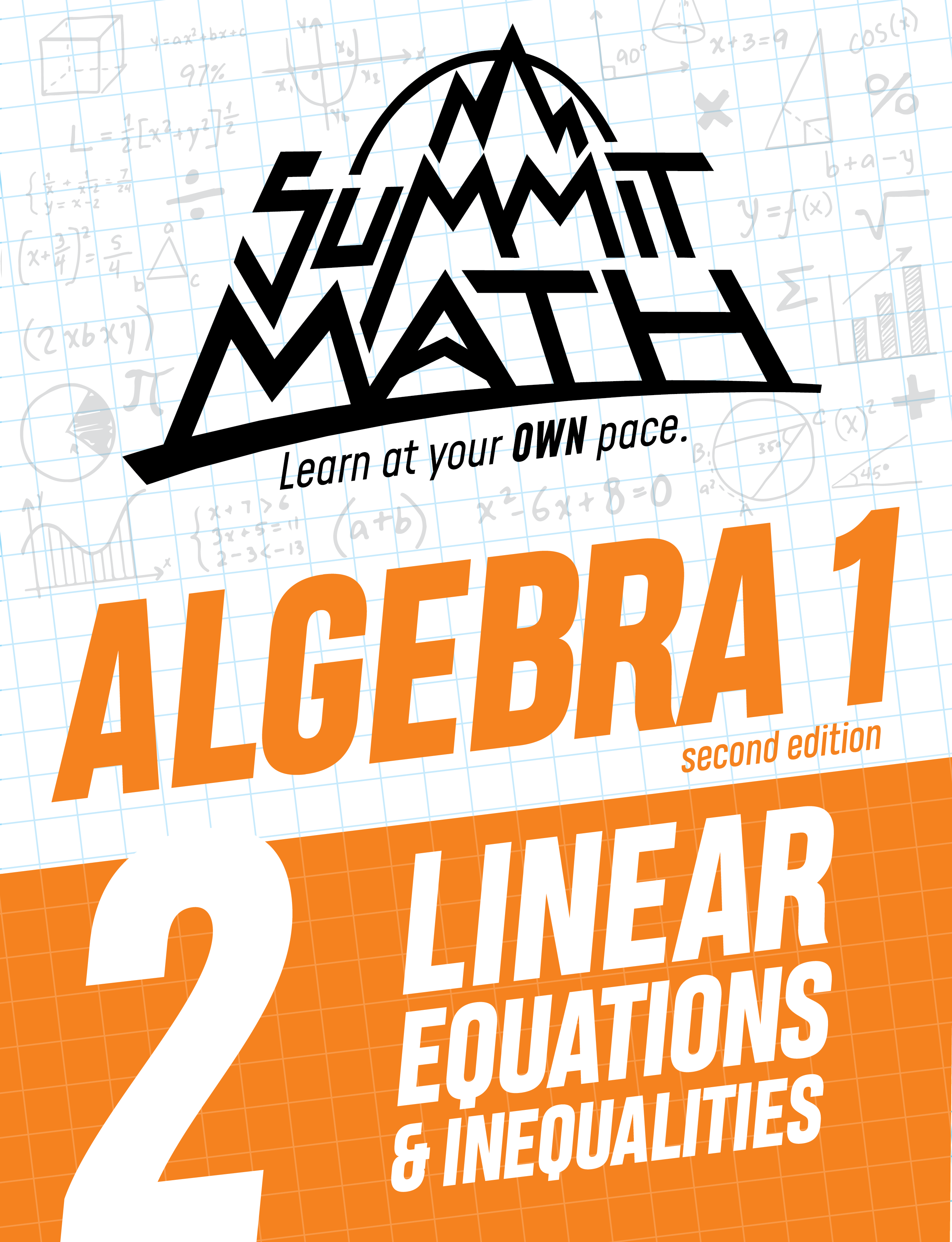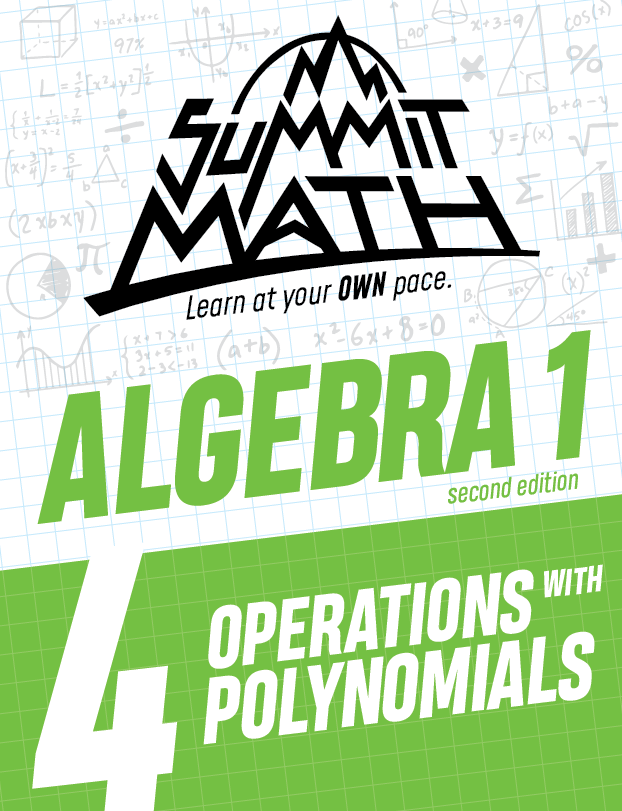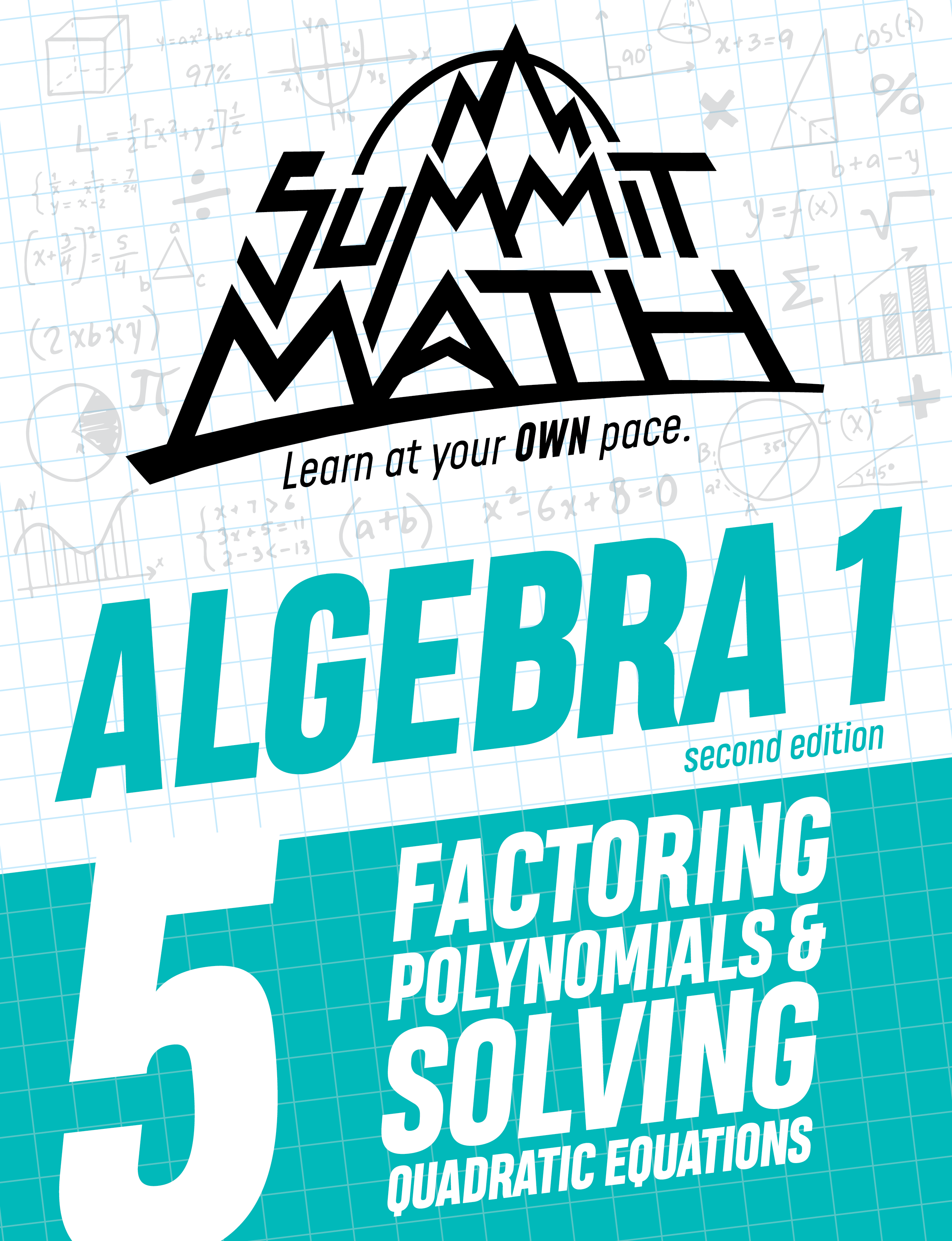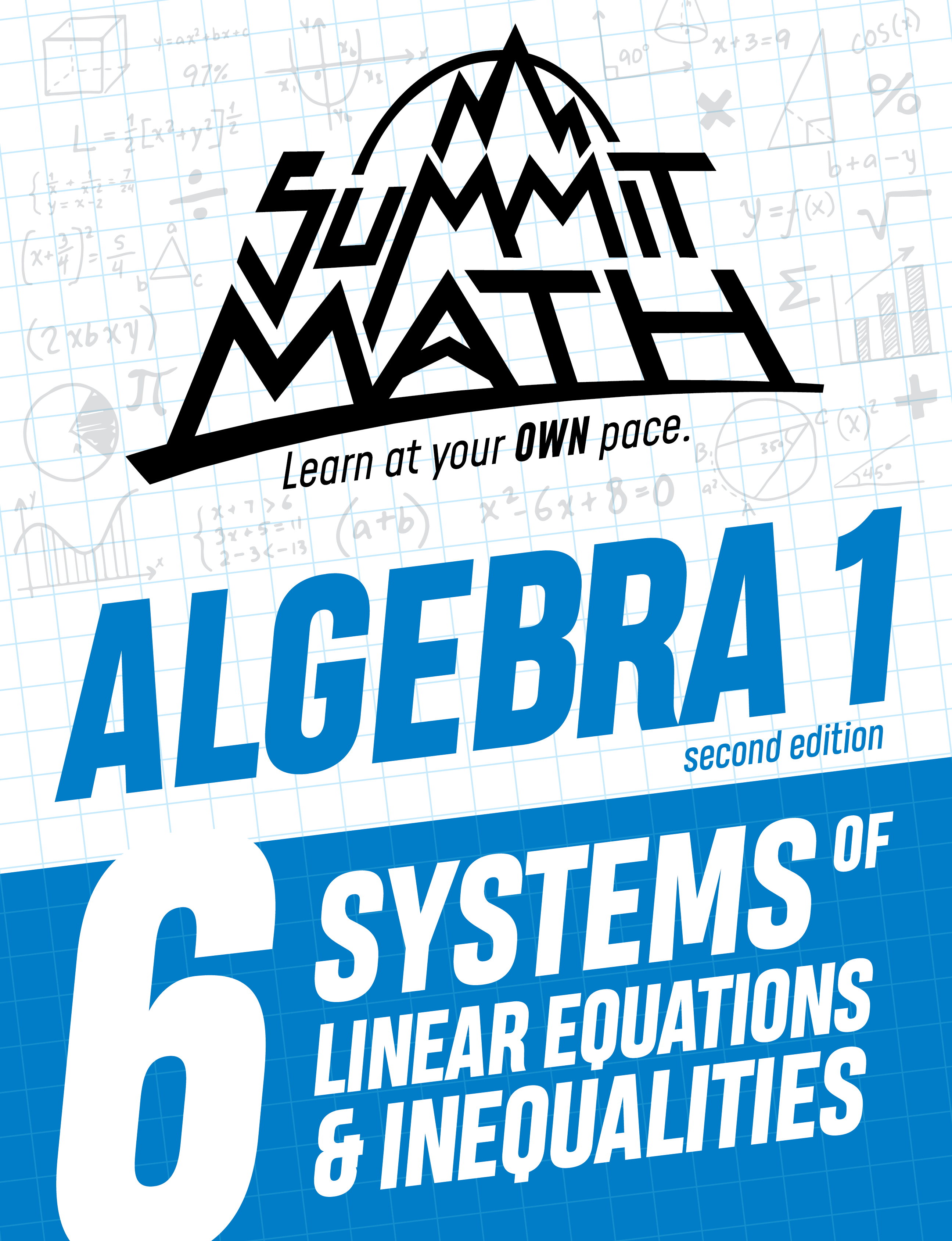## TEACHING VIDEOS

Subscribe to access videos that guide you through each book whenever you need it.

## LOOKING FOR ALGEBRA 1 TESTS?​

Click the link below to access the downloadable + printable Algebra 1 Review eBook. It contains labeled sections that align with each of the 7 books in the Algebra 1 Series. The Answer Key is located at the end of the eBook.# \$6.99

## CONTENTS​

Introduction to Percents

Percent Changes: Increases and Decreases

Writing Equations to Calculate Percents

Various Percent Scenarios

Introduction to Rates

Using Graphs to Calculate Rates

Rates in Equations

Cumulative Review

# \$9.99/mo.## CONTENTS​

Plotting Points on a Graph

Graphing a Line Using an Equation and a T-Chart

Graphing a Line Using its Intercepts

Constant Rates

The Slope of a Line

Writing a Line’s Equation in Slope-Intercept Form

Parallel and Perpendicular Lines

Scenarios that Involve Linear Equations

Linear Inequalities

Cumulative Review

# \$9.99/mo.## PROPERTIES OF EXPONENTS

100 pages​

CONTENTS

Introduction to Exponents

Multiplying Expressions with Exponents

Dividing Expressions with Exponents

Raising an Exponent to an Exponent

The Exponent of Zero

Negative Exponents

Evaluating Expressions with Exponents

Cumulative Review

# \$9.99/mo.# \$6.99

## 100 pages

CONTENTS

Introduction to Polynomials

Monomials, Binomials & Trinomials

Subtracting Polynomials

Multiplying Polynomials: Part 1

Writing Polynomials in Standard Form

Multiplying Polynomials: Part 2

When the Product of Binomials is a Binomial

Squaring a Binomial to Make a Perfect Square Trinomial

Polynomial Scenarios

Cumulative Review

# \$9.99/mo.# \$6.99

## ​

​CONTENTS

Review Multiplying Polynomials

Writing a Trinomial as a Product of Two Binomials

Factoring a Difference of Two Squares

Factoring a Perfect Square Trinomial

Using Factoring to Solve Equations

Scenarios that Involve Factoring

Using Factoring to Simplify Fractions

Introduction to Graphing Parabolas

Cumulative Review

# \$9.99/mo.# \$9.99/mo.

## CONTENTS

Introduction to Intersecting Lines

Using Equations to Find an Intersection Point

The Substitution Method

The Elimination Method

When Two Lines Do Not Intersect at One Point

Scenarios that Involve Systems of Equations

Systems of Linear Inequalities

More Scenarios that Involve Systems of Equations

Cumulative Review# \$6.99

## CONTENTS

Exponents and Roots

Comparing Rational and Irrational Numbers

How to Rationalize a Denominator

The Pythagorean Theorem

Cumulative Review

# \$9.99/mo.

## 8 books.  880+ pages.  2,100+ scenarios.